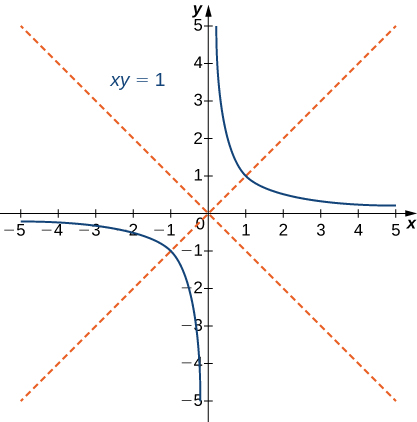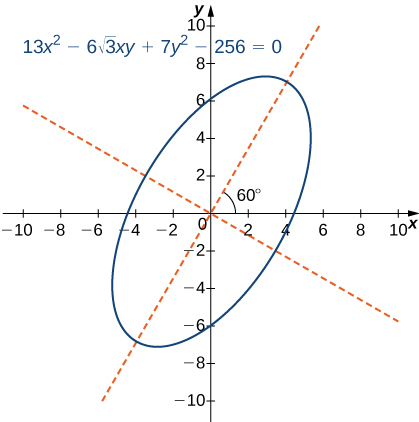# 1.5 Conic sections  (Page 9/23)

 Page 9 / 23
1. $4AC-{B}^{2}>0.$ If so, the graph is an ellipse.
2. $4AC-{B}^{2}=0.$ If so, the graph is a parabola.
3. $4AC-{B}^{2}<0.$ If so, the graph is a hyperbola.

The simplest example of a second-degree equation involving a cross term is $xy=1.$ This equation can be solved for y to obtain $y=\frac{1}{x}.$ The graph of this function is called a rectangular hyperbola as shown.Graph of the equation x y = 1 ; The red lines indicate the rotated axes.

The asymptotes of this hyperbola are the x and y coordinate axes. To determine the angle $\theta$ of rotation of the conic section, we use the formula $\text{cot}\phantom{\rule{0.2em}{0ex}}2\theta =\frac{A-C}{B}.$ In this case $A=C=0$ and $B=1,$ so $\text{cot}\phantom{\rule{0.2em}{0ex}}2\theta =\left(0-0\right)\text{/}1=0$ and $\theta =45\text{°}.$ The method for graphing a conic section with rotated axes involves determining the coefficients of the conic in the rotated coordinate system. The new coefficients are labeled ${A}^{\prime },{B}^{\prime },{C}^{\prime },{D}^{\prime },{E}^{\prime },\phantom{\rule{0.2em}{0ex}}\text{and}\phantom{\rule{0.2em}{0ex}}{F}^{\prime },$ and are given by the formulas

$\begin{array}{ccc}\hfill {A}^{\prime }& =\hfill & A\phantom{\rule{0.2em}{0ex}}{\text{cos}}^{2}\theta +B\phantom{\rule{0.2em}{0ex}}\text{cos}\phantom{\rule{0.2em}{0ex}}\theta \phantom{\rule{0.2em}{0ex}}\text{sin}\phantom{\rule{0.2em}{0ex}}\theta +C\phantom{\rule{0.2em}{0ex}}{\text{sin}}^{2}\theta \hfill \\ \hfill {B}^{\prime }& =\hfill & 0\hfill \\ \hfill {C}^{\prime }& =\hfill & A\phantom{\rule{0.2em}{0ex}}{\text{sin}}^{2}\theta -B\phantom{\rule{0.2em}{0ex}}\text{sin}\phantom{\rule{0.2em}{0ex}}\theta \phantom{\rule{0.2em}{0ex}}\text{cos}\phantom{\rule{0.2em}{0ex}}\theta +C\phantom{\rule{0.2em}{0ex}}{\text{cos}}^{2}\theta \hfill \\ \hfill {D}^{\prime }& =\hfill & D\phantom{\rule{0.2em}{0ex}}\text{cos}\phantom{\rule{0.2em}{0ex}}\theta +E\phantom{\rule{0.2em}{0ex}}\text{sin}\phantom{\rule{0.2em}{0ex}}\theta \hfill \\ \hfill {E}^{\prime }& =\hfill & \text{−}D\phantom{\rule{0.2em}{0ex}}\text{sin}\phantom{\rule{0.2em}{0ex}}\theta +E\phantom{\rule{0.2em}{0ex}}\text{cos}\phantom{\rule{0.2em}{0ex}}\theta \hfill \\ \hfill {F}^{\prime }& =\hfill & F.\hfill \end{array}$

The procedure for graphing a rotated conic is the following:

1. Identify the conic section using the discriminant $4AC-{B}^{2}.$
2. Determine $\theta$ using the formula $\text{cot}\phantom{\rule{0.2em}{0ex}}2\theta =\frac{A-C}{B}.$
3. Calculate ${A}^{\prime },{B}^{\prime },{C}^{\prime },{D}^{\prime },{E}^{\prime },\phantom{\rule{0.2em}{0ex}}\text{and}\phantom{\rule{0.2em}{0ex}}{F}^{\prime }.$
4. Rewrite the original equation using ${A}^{\prime },{B}^{\prime },{C}^{\prime },{D}^{\prime },{E}^{\prime },\phantom{\rule{0.2em}{0ex}}\text{and}\phantom{\rule{0.2em}{0ex}}{F}^{\prime }.$
5. Draw a graph using the rotated equation.

## Identifying a rotated conic

Identify the conic and calculate the angle of rotation of axes for the curve described by the equation

$13{x}^{2}-6\sqrt{3}xy+7{y}^{2}-256=0.$

In this equation, $A=13,B=-6\sqrt{3},C=7,D=0,E=0,$ and $F=-256.$ The discriminant of this equation is $4AC-{B}^{2}=4\left(13\right)\left(7\right)-{\left(-6\sqrt{3}\right)}^{2}=364-108=256.$ Therefore this conic is an ellipse. To calculate the angle of rotation of the axes, use $\text{cot}\phantom{\rule{0.2em}{0ex}}2\theta =\frac{A-C}{B}.$ This gives

$\begin{array}{cc}\hfill \text{cot}\phantom{\rule{0.2em}{0ex}}2\theta & =\frac{A-C}{B}\hfill \\ & =\frac{13-7}{-6\sqrt{3}}\hfill \\ & =-\frac{\sqrt{3}}{3}.\hfill \end{array}$

Therefore $2\theta ={120}^{\text{o}}$ and $\theta ={60}^{\text{o}},$ which is the angle of the rotation of the axes.

To determine the rotated coefficients, use the formulas given above:

$\begin{array}{ccc}\hfill {A}^{\prime }& =\hfill & A\phantom{\rule{0.2em}{0ex}}{\text{cos}}^{2}\theta +B\phantom{\rule{0.2em}{0ex}}\text{cos}\phantom{\rule{0.2em}{0ex}}\theta \phantom{\rule{0.2em}{0ex}}\text{sin}\phantom{\rule{0.2em}{0ex}}\theta +C\phantom{\rule{0.2em}{0ex}}{\text{sin}}^{2}\theta \hfill \\ & =\hfill & 13{\text{cos}}^{2}60+\left(-6\sqrt{3}\right)\phantom{\rule{0.2em}{0ex}}\text{cos}\phantom{\rule{0.2em}{0ex}}60\phantom{\rule{0.2em}{0ex}}\text{sin}\phantom{\rule{0.2em}{0ex}}60+7{\text{sin}}^{2}60\hfill \\ & =\hfill & 13{\left(\frac{1}{2}\right)}^{2}-6\sqrt{3}\left(\frac{1}{2}\right)\left(\frac{\sqrt{3}}{2}\right)+7{\left(\frac{\sqrt{3}}{2}\right)}^{2}\hfill \\ & =\hfill & 4,\hfill \\ \hfill {B}^{\prime }& =\hfill & 0,\hfill \\ \hfill {C}^{\prime }& =\hfill & A\phantom{\rule{0.2em}{0ex}}{\text{sin}}^{2}\theta -B\phantom{\rule{0.2em}{0ex}}\text{sin}\phantom{\rule{0.2em}{0ex}}\theta \phantom{\rule{0.2em}{0ex}}\text{cos}\phantom{\rule{0.2em}{0ex}}\theta +C\phantom{\rule{0.2em}{0ex}}{\text{cos}}^{2}\theta \hfill \\ & =\hfill & 13{\text{sin}}^{2}60+\left(-6\sqrt{3}\right)\phantom{\rule{0.2em}{0ex}}\text{sin}\phantom{\rule{0.2em}{0ex}}60\phantom{\rule{0.2em}{0ex}}\text{cos}\phantom{\rule{0.2em}{0ex}}60=7{\text{cos}}^{2}60\hfill \\ \hfill & =\hfill & {\left(\frac{\sqrt{3}}{2}\right)}^{2}+6\sqrt{3}\left(\frac{\sqrt{3}}{2}\right)\left(\frac{1}{2}\right)+7{\left(\frac{1}{2}\right)}^{2}\hfill \\ & =\hfill & 16,\hfill \\ \hfill {D}^{\prime }& =\hfill & D\phantom{\rule{0.2em}{0ex}}\text{cos}\phantom{\rule{0.2em}{0ex}}\theta +E\phantom{\rule{0.2em}{0ex}}\text{sin}\phantom{\rule{0.2em}{0ex}}\theta \hfill \\ & =\hfill & \left(0\right)\phantom{\rule{0.2em}{0ex}}\text{cos}\phantom{\rule{0.2em}{0ex}}60+\left(0\right)\phantom{\rule{0.2em}{0ex}}\text{sin}\phantom{\rule{0.2em}{0ex}}60\hfill \\ & =\hfill & 0,\hfill \\ \hfill {E}^{\prime }& =\hfill & \text{−}D\phantom{\rule{0.2em}{0ex}}\text{sin}\phantom{\rule{0.2em}{0ex}}\theta +E\phantom{\rule{0.2em}{0ex}}\text{cos}\phantom{\rule{0.2em}{0ex}}\theta \hfill \\ & =\hfill & \text{−}\left(0\right)\phantom{\rule{0.2em}{0ex}}\text{sin}\phantom{\rule{0.2em}{0ex}}60+\left(0\right)\phantom{\rule{0.2em}{0ex}}\text{cos}\phantom{\rule{0.2em}{0ex}}60\hfill \\ & =\hfill & 0,\hfill \\ \hfill {F}^{\prime }& =\hfill & F\hfill \\ & =\hfill & -256.\hfill \end{array}$

The equation of the conic in the rotated coordinate system becomes

$\begin{array}{}\\ \hfill 4{\left({x}^{\prime }\right)}^{2}+16{\left({y}^{\prime }\right)}^{2}& =\hfill & 256\hfill \\ \hfill \frac{{\left({x}^{\prime }\right)}^{2}}{64}+\frac{{\left({y}^{\prime }\right)}^{2}}{16}& =\hfill & 1.\hfill \end{array}$

A graph of this conic section appears as follows.Graph of the ellipse described by the equation 13 x 2 − 6 3 x y + 7 y 2 − 256 = 0 . The axes are rotated 60 ° . The red dashed lines indicate the rotated axes.

Identify the conic and calculate the angle of rotation of axes for the curve described by the equation

$3{x}^{2}+5xy-2{y}^{2}-125=0.$

The conic is a hyperbola and the angle of rotation of the axes is $\theta =22.5\text{°}.$

## Key concepts

• The equation of a vertical parabola in standard form with given focus and directrix is $y=\frac{1}{4p}{\left(x-h\right)}^{2}+k$ where p is the distance from the vertex to the focus and $\left(h,k\right)$ are the coordinates of the vertex.
• The equation of a horizontal ellipse in standard form is $\frac{{\left(x-h\right)}^{2}}{{a}^{2}}+\frac{{\left(y-k\right)}^{2}}{{b}^{2}}=1$ where the center has coordinates $\left(h,k\right),$ the major axis has length 2 a, the minor axis has length 2 b , and the coordinates of the foci are $\left(h±c,k\right),$ where ${c}^{2}={a}^{2}-{b}^{2}.$
• The equation of a horizontal hyperbola in standard form is $\frac{{\left(x-h\right)}^{2}}{{a}^{2}}-\frac{{\left(y-k\right)}^{2}}{{b}^{2}}=1$ where the center has coordinates $\left(h,k\right),$ the vertices are located at $\left(h±a,k\right),$ and the coordinates of the foci are $\left(h±c,k\right),$ where ${c}^{2}={a}^{2}+{b}^{2}.$
• The eccentricity of an ellipse is less than 1, the eccentricity of a parabola is equal to 1, and the eccentricity of a hyperbola is greater than 1. The eccentricity of a circle is 0.
• The polar equation of a conic section with eccentricity e is $r=\frac{ep}{1±e\phantom{\rule{0.2em}{0ex}}\text{cos}\phantom{\rule{0.2em}{0ex}}\theta }$ or $r=\frac{ep}{1±e\phantom{\rule{0.2em}{0ex}}\text{sin}\phantom{\rule{0.2em}{0ex}}\theta },$ where p represents the focal parameter.
• To identify a conic generated by the equation $A{x}^{2}+Bxy+C{y}^{2}+Dx+Ey+F=0,$ first calculate the discriminant $D=4AC-{B}^{2}.$ If $D>0$ then the conic is an ellipse, if $D=0$ then the conic is a parabola, and if $D<0$ then the conic is a hyperbola.

What do we mean by Asian tigers
Dm me I will tell u
Shailendra
Hi
Aeesha
hi
Pixel
What is Average revenue
KEMZO
How are u doing
KEMZO
it is so fantastic
metasebia
what's a demand
it is the quantity of commodities that consumers are willing and able to purchase at particular prices and at a given time
Munanag
quantity of commodities dgat consumers are willing to pat at particular price
Omed
demand depends upon 2 things 1wish to buy 2 have purchasing power of that deserving commodity except any from both can't be said demand.
Bashir
Demand is a various quantity of a commodities that a consumer is willing and able to buy at a particular price within a given period of time. All other things been equal.
Vedzi
State the law of demand
Vedzi
The desire to get something is called demand.
Mahabuba
what is the use of something should pay for its opportunity foregone to indicate?
Why in monopoly does the firm maximize profits when its marginal revenue equals marginal cost
different between economic n history
If it is known that the base change of RM45 million, the statutory proposal ratio of 7 per cent, and the public cash holding ratio of 5 per cent, what is the proposed ratio of bank surplus to generate a total deposit of RM 300 million?
In a single bank system, a bank can create a deposit when it receives a new deposit in cash. If a depositor puts a cash deposit of RM10,000 into the bank, assume the statutory reserve requirement is 7% and the bank adopts a surplus reserve of 8%. a. Calculate the amount of deposits made at the end o
Jeslyne
the part of marginal revenue product curve lies in the _ stage of production is called form demand curve for variable input.
The cost associated with the inputs owned by the farmer is termed as
Bashir
the cost associated with inputs owned by the farmer is termed as ____
Bashir
why do we study economic
we study economics to know how to manage our limited resources
Eben
တစ်ဦးကျဝင်​ငွေ
myo
we study economics the know how to use our resources and where to put it
Mamoud
what is end
Nwobodo
we study economics to make rational decision
Gloria
we study economics only to know how to effectively and efficiently allocate our limited resource in other to meet our unlimited wants
Kpegba
We study economics inorder for us to know the difference of the needs and wants and aslo how to use the limited resources that are available
Bongani
who is the father of economy
ibrahim
Somnath
professor Lionel Robins
Abraham
albert
mariginal utility is finalized by who?
Barsharani
marshall
Laila
Mamoud
Bongani
Veronica
Omed
why we study economics
what is equilibrium price?
This is the price In which quantity demanded is equal to the quantity supplied.
Binta
what is the principle of demand
Udoh
is when the price of two item is equal
Mamoud
is the market price at which the demand curve and supply curve of particular commodity interest.
Kpegba
can we say that without macroeconomics,microeconomics can succeed? and why?
Kpegba
equilibrium price is when prices are equal
Ramon
equilibrium price is a point at which demand and supply curve meet
Vedzi
please can you give us the correct answer after the lesson to be compared to our answers
in what?
ibrahim
why economics is the real life subject
Kitojo
because it is subjected to human decisions
Kpegba
why might an increase in money national income not necssarily lead to an increase in the standards of living
Multin
pls,who is a legal tender.can you explain well
We think, that the legal tender is a form of payment of a debt or anything related, but which is not necessarily money. that can be bank notes, or coins for instance. but the bottom line is the legal tender is required to be recognized by the law, but it varies according to the jurisdiction.
Pierre
Thanks
Mary
Is it something like cheque
Mary
legal tender is anything that can be accepted for payment within a country
Tyson
good
Philo
is Something legally accepted in a particular place
Mamoud
Who is a liquidator?
pls,who is a legal tender
Mary
We think, that the legal tender is a form of payment of a debt or anything related, but which is not necessarily money. that can be bank notes, or coins for instance. but the bottom line is the legal tender is required to be recognized by the law, but it varies according to the jurisdiction.
Pierre
s and D are the only in the firm.the face following playoff D gain: large budget. small Bud 30billon. 0 zero 70billon. 50milln s gains large budg. small bud 20millon. 0zero 30. 40
in a comparison of the stages of meiosis to the stage of mitosis, which stages are unique to meiosis and which stages have the same event in botg meiosis and mitosis
can you provide the details of the parametric equations for the lines that defince doubly-ruled surfeces (huperbolids of one sheet and hyperbolic paraboloid). Can you explain each of the variables in the equations?

#### Get Jobilize Job Search Mobile App in your pocket Now!By Brooke DelaneyBy Stephanie RedfernBy Mary MateraBy OpenStaxBy OpenStaxBy OpenStaxBy Steve GibbsBy OpenStaxBy Marion CabalfinBy Richley Crapo